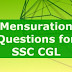## Most Important Mensuration Questions for SSC CGL Tier-2 (set - 1)

1. A cylindrical tank of diameter 35 cm is full of water. If 11 litres of water is drawn off, the water level in the tank will drop by-
A.21/2 cm
B.90/7 cm
C.14 cm
D.80/7 cm.

2.19. The height of a conical tank is 60 cm and the diameter of its base is 64 cm. The cost of painting it from outside at the rate of Rs. 35 per sq m is-
A.Rs. 52.00 approx
B.Rs. 39.20 approx
C.Rs. 35.20 approx
D.Rs. 23.94 approx

3. A solid metallic cone of height 10 cm, radius of base 20 cm is melted to make spherical balls each of 4 cm diameter. How many such balls can be made?
A.25
B.75
C.50
D.125

4. The area of a circle is 38.5 cm2 Its circumference in cm is (use π = 22/7)
A.22
B.24
C.26
D.32

5. The breadth of a rectangular hall is three-fourths of its length. If the area of the floor is 768 sq m, then the difference between the length and breadth of the hall is-
A.8 m
B.12 m
C.24 m
D.32 m

6. A metallic hemisphere is melted and recast in the shape of a cone with the same base radius (R) as that of the hemisphere. If H is the height of the cone
A.H=2R
B.H=2/3R
C.H=√3R
D.B=3R

7. A conical vessel whose internal radius is 12 cm and height 50 cm is full of liquid. The contents are emptied into a cylindrical vessel with radius (internal) 10 cm. The height to which the liquid rises in the cylindrical vessel is-
A.25 cm
B.20 cm
C.24 cm
D.22 cm

8. Two right circular cylinders of equal volume have their heights in the ratio 1:2. The ratio of their radii is:
A.√(2:1)
B.2:1
C.1:2
D.1:4

9. If the length of the diagonal AC of a square ABCD is 5.2 cm, then the area of the square is-
A.15.12 cm2
B.13.52 cm2
C.12.62 cm2
D.10.00 cm2

10. If diagonal of a cube is 12 cm, then its volume in cubic cm is-
A.8
B.12
C.24
D.3√2

11. The length and breadth of a rectangular field are in the ratio 3:2. If the perimeter of the field is 80 m, its breadth (in metres) is-
A.18
B.16
C.10
D.24

12. The radius of a circular wheel is 1.75 m. The number of revolutions it will make in travelling 11 km is-(use π=22/7)
A.800
B.900
C.1000
D.1200

13. If the radius of a sphere is increased by 2 cm, its surface area is increased by 352 cm2. The radius of sphere before change is(use π = 22/7)
A.3 cm
B.4 cm
C.5 cm
D.6 cm

14. The length of the diagonal of a square is ‘a’cm. Which of the following represents the area of the square (in sq. cm.)?
A.2a
B.a/√2
C.a2/2
D.a2/4

15. If the volumes of two cubes are in the ratio 27:1, the ratio of their is-
A.3:1
B.27:1
C.1:3
D.1:27

16. The sides of a rectangular plot are in the ratio 5:4 and its area is equal to 500 sq.m. The perimeter of the plot is-
A.80 m
B.100 m
C.90 m
D.95 m

17. The radius of a wheel is 21 cm. How many revolution will t make in travelling 924 metres?
A.7
B.11
C.200
D.700

18. The edges of a cuboid are in the ratio 1:2:3 and its surface area is 88 cm2. The volume of the cuboid is-
A.120 cm cube
B.64 cm cube
C.48 cm cube
D.24 cm cube

19. The area of a sector of a circle of radius 5 cm, formed by an arc of length 3.5 cm is-
A.8.5 cm sq
B.8.75 cm sq
C.7.75 cm sq
D.7.50 cm sq

20. Find the length of the largest rod that can be placed in a room 16 m long, 12 m broad and 32/3 m high
A.23 m
B.68 m
C.68/3 m
D.67/3 m

21. In a swimming pool measuring 90 m by 40 m, 150 men take a dip. If the average displacement of water by a man is 8 cubic metres, what will be the rise in water level?
A. 33.33 cm
B. 30 cm
C. 20 cm
D. 25 cm

22. A square is inscribed in a circle of radius 8 cm. The area of the square is
A. 16 cm sq
B. 64 cm sq
C. 128 cm sq
D. 148 cm sq

23. The biggest possible circle is inscribed in a rectangle of length 16 cm and breadth 6 cm. Then its area is
A. 3p cm sq
B. 4p cm sq
C. 5p cm sq
D. 9p cm sq

24. If the diagonal of a square is doubled, then its area will be
A. three times
B. four times
C. same
D.none of these

25. A metal pipe of negligible thickness has radius 21 cm and length 90 cm. The outer curved surface area of the pipe in square cm is
A. 11880
B. 11680
C. 11480
D. 10080

1. D     2. D      3. D     4. A      5. A
6. A     7. C       8. A     9. B     10. A
11.B   12. C     13. D    14. C    15. A
16.C   17. D     18. C    19. B    20. C
21.A   22. C      23. D   24. B     25. A

Most Important Mensuration Questions for SSC CGL Tier-2 (set - 1)Reviewed by Admin on 10:57:00 PM Rating: 5